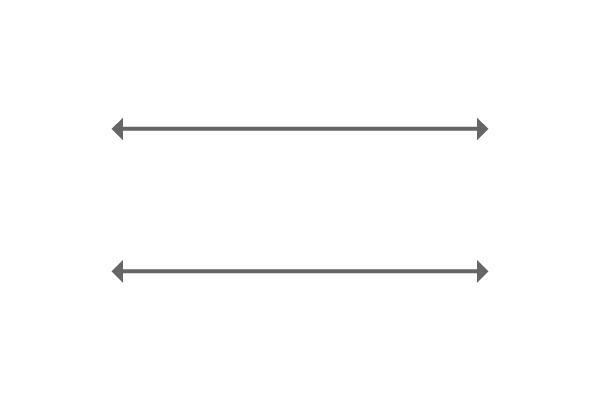# Parallel lines

Two or more straight lines which do not intersect each other at any point on the plane are called parallel lines.Parallel lines are straight lines basically and they appear on a plane side by side. They never intersect each other anywhere on a plane because of having same angle. Therefore, if two or more straight lines have same angle, then they do not meet each other.

Similarly, the vertical distance between opposite points of them is always same due to the parallelism of the parallel lines.

## Example$\overleftrightarrow{AB}$ and $\overleftrightarrow{CD}$ are two straight lines and they are extended infinitely but they do not intersect each other even though they are appearing side by side.

They maintain equal distance between them at all their opposite points. The reason for this is the angles of both straight lines are same.

The straight lines $\overleftrightarrow{AB}$ and $\overleftrightarrow{CD}$ are called parallel lines.

### Representation

A double vertical line ($\|$) is used to represent parallelism of the lines mathematically.

The parallel symbol is displayed between straight lines to express that they both are parallel lines.

$\overleftrightarrow{AB} \,\, \| \,\, \overleftrightarrow{CD}$

It is read as $\overleftrightarrow{AB}$ parallel to the $\overleftrightarrow{CD}$.

Similarly, it can also be displayed in other way.

$\overleftrightarrow{CD} \,\, \| \,\, \overleftrightarrow{AB}$

It is read as $\overleftrightarrow{CD}$ parallel to the $\overleftrightarrow{AB}$.

Latest Math Topics
Jun 26, 2023
Jun 23, 2023

Latest Math Problems
Jul 01, 2023
Jun 25, 2023
###### Math Questions

The math problems with solutions to learn how to solve a problem.

Learn solutions

Practice now

###### Math Videos

The math videos tutorials with visual graphics to learn every concept.

Watch now

###### Subscribe us

Get the latest math updates from the Math Doubts by subscribing us.